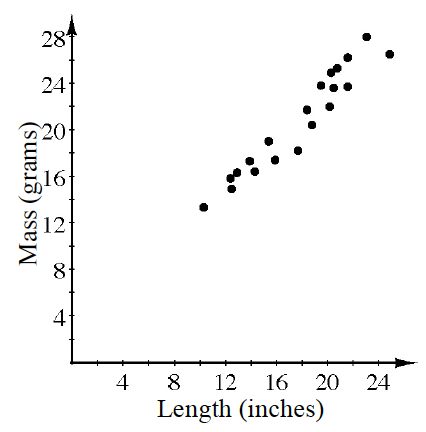### Home > CCA2 > Chapter A > Lesson A.1.3 > ProblemA-40

A-40.

The graph at right shows a comparison of the length of several gold chain necklaces (including the clasp) to the total mass.

1. Write an equation for the line of best fit.

Hold a straight-edge up to the graph to create a line and estimate the slope and $y$-intercept.

$y = x + 2$ is one possibility.

2. Based on your equation, what would you expect to be the mass of a $26$ inch chain?

Use the rule you wrote in part (a).
Which variable represents mass, $x$ or $y$?

About $28$ grams.